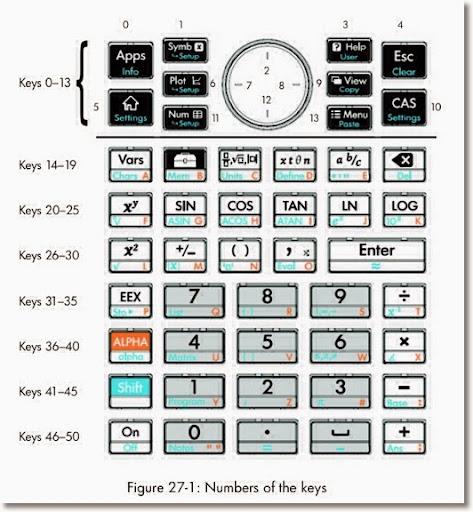## Tuesday, November 5, 2013

### HP Prime Programming Tutorial #3: WHILE, INPUT, KILL, REPEAT, GETKEY

This tutorial is going to cover a lot, each with some new programming commands in this series. I hope you are ready for the intensity. :)

WHILE, INPUT, KILL

HP Prime Program: TARGET. TARGET is a game where you provide a guess to get a desired number. If you miss, the calculator will tell you if number is higher and lower. At the end of the game, the calculator gives you how may picks you needed to get the target number.

WHILE: Repeat a number of commands while a specific condition is test.

WHILE condition is true DO
commands
END;

Access: Tmplt, 3. Loop, 5. WHILE

Caution: Watch your ENDs! Make sure an END is with each loop and the program itself. Press the soft key Check to check your work.

INPUT: Creates an input screen for variables. On the HP Prime, the input can asked for more than one input. TARGET demonstrates INPUT with one prompt.

One Variable:
INPUT(variable, "title", "label", "help text")
Multi-Variable:
INPUT(list of variables, "title", list of "labels", list of "help text")

Note: Pressing Cancel will store a 0 in variable. You may include code of what to do if the user presses Cancel, but it is not required.

Access: Cmds, 6. I/O, 5. INPUT

KILL: Terminates program execution. Nothing dies, I promise.

Access: Tmplt. 1. Block, 3. KILL

Program:
EXPORT TARGET()
BEGIN
LOCAL C:=0, N:=RANDINT(1,20), G:=-1;
WHILE G≠N DO
C:=C+1;
INPUT(G,"Guess?","GUESS:","1 - 20");

IF G==0 THEN
KILL;
END;

IF G < N THEN
MSGBOX("Higher");
END;

IF G > N THEN
MSGBOX("Lower");
END;

END;

MSGBOX("Correct! Score: "+C);

END;

Try it and of course, you can adjust the higher limit. Here is some thing for you to try with TARGET:

1. Add a limited amount of guesses.
2. Can you display the list of guesses?

REPEAT

ULAM Algorithm: take an integer n. If n is even, divide it by 2. If n is odd, multiply it by 3 and add 1. ULAM counts how many steps it takes to get n to 1.

REPEAT:

Access: Tmplt, 3. Loop, 6. REPEAT

Featured:
CONCAT(list1, list2): Melds list1 and list2 into one.

Access: Toolbox, Math, 6. List, 4. Concatenate

EXPORT ULAM(N)
BEGIN
LOCAL C:=1, L0:={N};

REPEAT

IF FP(N/2)==0 THEN
N:=N/2;
ELSE
N:=3*N+1;
END;

C:=C+1;
L0:=CONCAT(L0,{N});
UNTIL N==1;

MSGBOX("NO. OF STEPS="+C);
RETURN L0;
END;

Examples:

ULAM(5) returns:
Message Box: "NO. OF STEPS=6"
List: {5, 16, 8, 4, 2, 1}

ULAM(22) returns:
Message Box: "NO. OF STEPS=16"
List: {22, 11, 34, 17, 52, 26, 13, 40, 20, 10, 5, 16, 8, 4, 2, 1}

GETKEY

The next section will introduce a super-important command, GETKEY. We will be working with GETKEY over the entire series.

The Program KEYNO: The person presses key presses. Which each key press, the code returns to the terminal screen. The program terminates when the Enter key is pressed.

GETKEY: Returns the key code of last key pressed. The Prime's key map is below. (Picture is from the HP Prime User's Guide)

Access: Cmds, 6. I/O, 4. GETKEYEXPORT KEYNO()
BEGIN
LOCAL K;
PRINT();
PRINT("Press any key to get its code.");
PRINT("Press Enter to exit.");

REPEAT
K:=GETKEY;
IF K ≥ 0 THEN
PRINT(K);
END;
UNTIL K==30;

END;

Example Key Codes:
33: 8 key
2: up
7: left
8: right
12: down
50: plus
45: minus

This concludes Part 3. Again, it can't be said enough, thanks for all the comments and compliments. And until next time,

Eddie

This blog is property of Edward Shore. 2013

### Casio fx-5000F: Auto Formulas

Casio fx-5000F:   Auto Formulas The formula listing can apply to (almost) any calculator that can handle formula programming. In November, t...